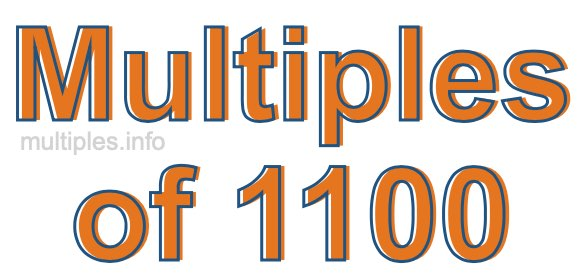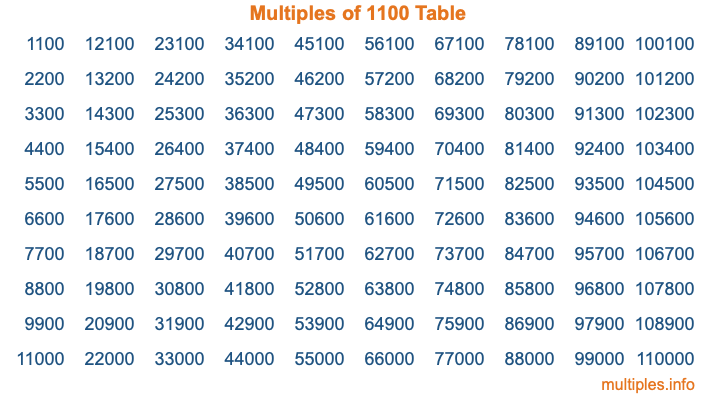Multiples of 1100Welcome to the Multiples of 1100 page. Here we will first teach you everything you will ever need to know about the multiples of 1100, and then give you a study guide summary of everything we taught you to make sure you remember it all. Use this page to look up facts and learn information about the multiples of 1100. This page will make you a multiples of one thousand one hundred expert!

Definition of Multiples of 1100
Multiples of 1100 are all the numbers that when divided by 1100 equal an integer. Each of the multiples of 1100 are called a multiple. A multiple of 1100 is created by multiplying 1100 by an integer.

Therefore, to create a list of multiples of 1100, you start with 1 multiplied by 1100, then 2 multiplied by 1100, then 3 multiplied by 1100, and so on for as long as you want. Thus, the list of the first five multiples of 1100 is 1100, 2200, 3300, 4400, and 5500. To see a larger list of multiples of 1100, see the printable image of Multiples of 1100 further down on this page. We also have a category where you can choose any nth multiple of 1100.

Multiples of 1100 Checker
The Multiples of 1100 Checker below checks to see if any number of your choice is a multiple of 1100. In other words, it checks to see if there is any number (integer) that when multiplied by 1100 will equal your number. To do that, we divide your number by 1100. If the the quotient is an integer, then your number is a multiple of 1100.

Is  a multiple of 1100?

Least Common Multiple of 1100 and ...
A Least Common Multiple (LCM) is the lowest multiple that two or more numbers have in common. This is also called the smallest common multiple or lowest common multiple and is useful to know when you are adding our subtracting fractions. Enter one or more numbers below (1100 is already entered) to find the LCM.

Check out our LCM Calculator if you need more details about the Least Common Multiple or if you need the LCM for different numbers for adding and subtraction fractions.

nth Multiple of 1100
As we stated above, 1100 is the first multiple of 1100, 2200 is the second multiple of 1100, 3300 is the third multiple of 1100, and so on. Enter a number below to find the nth multiple of 1100.

th multiple of 1100

Multiples of 1100 vs Factors of 1100
1100 is a multiple of 1100 and a factor of 1100, but that is where the similarities end. All postive multiples of 1100 are 1100 or greater than 1100. All positive factors of 1100 are 1100 or less than 1100.

Below is the beginning list of multiples of 1100 and the factors of 1100 so you can compare:

Multiples of 1100: 1100, 2200, 3300, 4400, 5500, etc.

Factors of 1100: 1, 2, 4, 5, 10, 11, 20, 22, 25, 44, 50, 55, 100, 110, 220, 275, 550, 1100

As you can see, the multiples of 1100 are all the numbers that you can divide by 1100 to get a whole number. The factors of 1100, on the other hand, are all the whole numbers that you can multiply by another whole number to get 1100.

It's also interesting to note that if a number (x) is a factor of 1100, then 1100 will also be a multiple of that number (x).

Multiples of 1100 vs Divisors of 1100
The divisors of 1100 are all the integers that 1100 can be divided by evenly. Below is a list of the divisors of 1100.

Divisors of 1100: 1, 2, 4, 5, 10, 11, 20, 22, 25, 44, 50, 55, 100, 110, 220, 275, 550, 1100

The interesting thing to note here is that if you take any multiple of 1100 and divide it by a divisor of 1100, you will see that the quotient is an integer.

Multiples of 1100 Table
Below is an image of the first 100 multiples of 1100 in a table. The table is in chronological order, column by column. The first column has the first ten multiples of 1100, the second column has the next ten multiples of 1100, and so on.The Multiples of 1100 Table is also referred to as the 1100 Times Table or Times Table of 1100. You are welcome to print out our table for your studies.

Negative Multiples of 1100
Although not often discussed or needed in math, it is worth mentioning that you can make a list of negative multiples of 1100 by multiplying 1100 by -1, then by -2, then by -3, and so on, to get the following list of negative multiples of 1100:

-1100, -2200, -3300, -4400, -5500, etc.

Multiples of 1100 Summary
Below is a summary of important Multiples of 1100 facts that we have discussed on this page. To retain the knowledge on this page, we recommend that you read through the summary and explain to yourself or a study partner why they hold true.

There are an infinite number of multiples of 1100.

A multiple of 1100 divided by 1100 will equal a whole number.

1100 divided by a factor of 1100 equals a divisor of 1100.

The nth multiple of 1100 is n times 1100.

The largest factor of 1100 is equal to the first positive multiple of 1100.

1100 is a multiple of every factor of 1100.

1100 is a multiple of 1100.

A multiple of 1100 divided by a divisor of 1100 equals an integer.

1100 divided by a divisor of 1100 equals a factor of 1100.

Any integer times 1100 will equal a multiple of 1100.

Multiples of a Number
Here you can get the multiples of another number, all with the same attention to detail as we did for multiples of 1100 on this page.

Multiples of
Multiples of 1101
Did you find our page about multiples of one thousand one hundred educational? Do you want more knowledge? Check out the multiples of the next number on our list!